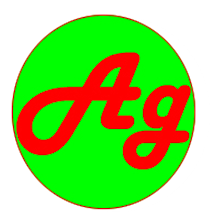# Syllabus - ENGINEERING MATHEMATICS II SH 451 - for TU IOE All BE first year second semester

ENGINEERING MATHEMATICS II
SH 451

Lecture: 3                                                                                                                  Year: 1
Tutorial: 2                                                                                                                 Part: II
Practical

Course Objectives:            i)        To develop the skill of solving differential equations and to provide knowledge of vector algebra and calculus
ii)       To make students familiar with calculus of several variables
and infinite series

1.          Calculus of two or more variables                                                                        (6 hours)
1.1.       Introduction: limit and continuity
1.2.       Partial derivatives
1.2.1.     Homogeneous function, Euler’s theorem for the function of
two and three variables
1.2.2.     Total derivatives
1.3.       Extrema of functions of two and three variables; Lagrange’s Multiplier

2.          Multiple Integrals                                                                                                     (6 hours)
2.1.       Introduction
2.2.       Double integrals in Cartesian and polar form; change of order of integration
2.3.       Triple integrals in Cartesian, cylindrical and spherical coordinates;
2.4.       Area and volume by double and triple integrals

3.          Three Dimensional Solid Geometry                                                                  (11 hours)
3.1.       The straight line; Symmetric and general form
3.2.       Coplanar lines
3.3.       Shortest distance
3.4.       Sphere
3.5.       Plane Section of a sphere by planes
3.6.       Tangent Planes and lines to the spheres
3.7.       Right circular cone
3.8.       Right circular cylinder

4.          Solution of Differential Equations in Series and Special Functions                    (9 hours)
4.1.       Solution of differential equation by power series method
4.2.       Legendre’s equation
4.3.       Legendre polynomial function; Properties and applications.
4.4.       Bessel’s equation
4.5.       Bessel’s function of first and second kind. Properties and applications

5.          Vector Algebra and Calculus                                                                                (8 hours)
5.1.       Introduction
5.2.       Two and three dimensional vectors
5.3.       Scalar products and vector products
5.4.       Reciprocal System of vectors
5.5.       Application of vectors: Lines and planes
5.6.       Scalar and vector fields
5.7.       Derivatives – Velocity and acceleration
5.8.       Directional derivatives

6.          Infinite Series                                                                                                             (5 hours)
6.1.       Introduction
6.2.       Series with positives terms
6.3.       convergence and divergence
6.4.       Alternating series. Absolute convergence
6.5.       Radius and interval of convergence

Reference books:
1.       Erwin Kreyszig, Advanced Engineering Mathematics , John Wiley and Sons Inc
2.       Thomas, Finney, Calculus and Analytical geometry Addison- Wesley
3.       M. B. Singh, B. C. Bajrachrya,  Differential calculus, Sukunda  Pustak Bhandar,Nepal
4.       M. B. Singh, B. C. Bajrachrya,  A text book of Vectors, Sukunda  Pustak Bhandar,Nepal
5.       M. B. Singh,  S. P. Shrestha, Applied Mathematics,
6.       G.D. Pant, G. S. Shrestha, Integral Calculus and Differential Equations, Sunila Prakashan,Nepal
7.       Y. R. Sthapit, B. C. Bajrachrya,  A text book of Three Dimensional Geometry, Sukunda  Pustak Bhandar,Nepal
8.       Santosh Man Maskey, Calculus, Ratna Pustak Bhandar, Nepal
Evaluation Scheme:
The questions will cover all the chapters in the syllabus. The evaluation scheme will be as indicated in the table below:

 Chapter Hours Mark distribution * 1. 06 10 2. 06 10 3. 11 20 4. 09 15 5. 08 15 6. 05 10 Total 45 80
* There may be minor deviation in marks distribution.

###### Share To: